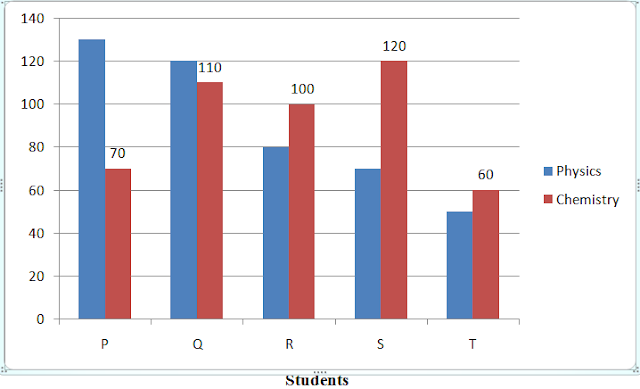New Students Offer - Use Code HELLO

# Data Interpretation - Question Set 10

Directions (1 - 5): Study the following bar graph carefully to answer the questions.
Five students namely Param, Qartar, Rasheed, Sultan and Tango are termed as P, Q, R, S and T.
Marks obtained by them in Physics and Chemistry :-1. Marks obtained by Sultan in Chemistry is what percent of the total marks obtained by all the students in Chemistry?
a) 26                            b) 28.5                         c) 35
d) 31.5                         e) 22

2. If the marks obtained by Tango in Physics were increased by 14% of the original marks, what would be his new approximate percentage in Physics if the maximum marks in Physics were 140?
a) 57                            b) 32                            c) 38
d) 48                            e) 41

3. Fill in the blank space in order to make the sentence correct as per the given information. Total marks obtained by Tango in both the subjects together is more than the marks obtained by
a) Qartar in Chemistry        b) Rasheed in Physics             c) Sultan in Chemistry
d) Param in Physics             e) Rasheed in both the subjects together

4. What is the respective ratio between the total marks obtained by Param in Physics and Chemistry together to the total marks obtained by Tango in Physics and Chemistry together?
a) 3 : 2                         b) 20 : 11                         c) 5 : 3
d) 2 : 1                         e) None of these

5. What is the respective ratio between the total marks obtained by Qartar and Sultan together in Chemistry to the total marks obtained by Param and Rasheed together in Physics?
a) 23 : 25                     b) 23 : 21                     c) 17 : 19

d) 17 : 23                     e) None of these

Solution – 1 (Option A)
Required percentage mark = 120 / 90 + 110 + 100 + 120 + 60 × 100
120 / 460 × 100 = 26%

Solution – 2 (Option E)
New marks of T in physics = 114 / 100 × 50 = 57
T’s new percentage =  57 / 140× 100 =  41

Solution – 3 (Option B)
Marks obtained by Tango in both subjects together is more than the marks obtained by                         Rasheed in Physics.

Solution – 4 (Option D)
Required ratio = 130 + 70 : 50 + 60
= 200 : 110                  20: 11

Solution – 5 (Option B)
Required ratio = 110 + 120 : 130 + 80

= 230 : 210                  23 : 21